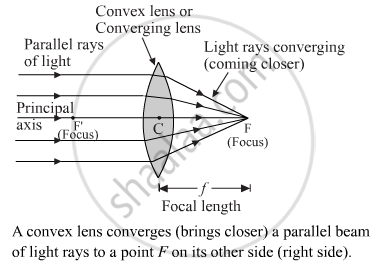Share

# Explain with the Help of a Diagram, Why the Convex Lens is Also Called a Converging Lens. - CBSE Class 10 - Science

ConceptRefraction of Light Image Formation by Lenses - Convex Lens

#### Question

Explain with the help of a diagram, why the convex lens is also called a converging lens.

#### Solution

A convex lense is outwardly curved and causes the light to pass through it and converge or concentrate to a point. Think of a magnifying glass that is used to burn something. The light that passes through it concentrates to a point, and this convergence is used to burn things. See the diagram given below.Is there an error in this question or solution?

#### Video TutorialsVIEW ALL 

Solution Explain with the Help of a Diagram, Why the Convex Lens is Also Called a Converging Lens. Concept: Refraction of Light - Image Formation by Lenses - Convex Lens.
S